Courses

# Test: Motion In Plane - Introduction To Vectors

## 10 Questions MCQ Test Physics Class 11 | Test: Motion In Plane - Introduction To Vectors

Description
This mock test of Test: Motion In Plane - Introduction To Vectors for Class 11 helps you for every Class 11 entrance exam. This contains 10 Multiple Choice Questions for Class 11 Test: Motion In Plane - Introduction To Vectors (mcq) to study with solutions a complete question bank. The solved questions answers in this Test: Motion In Plane - Introduction To Vectors quiz give you a good mix of easy questions and tough questions. Class 11 students definitely take this Test: Motion In Plane - Introduction To Vectors exercise for a better result in the exam. You can find other Test: Motion In Plane - Introduction To Vectors extra questions, long questions & short questions for Class 11 on EduRev as well by searching above.
QUESTION: 1

### Find the area of parallelogram formed by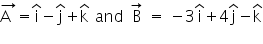Solution: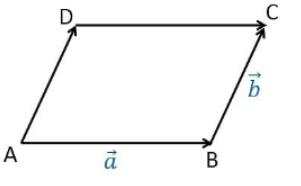Area of Parallelogram = breadth x height⟂ = |(A x B)|

Here, A = i - j + k

And,  B = -3i + 4j -k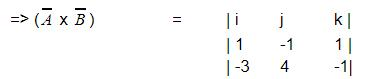= i (1-4) -j (-3+1) +k (4-3)

=> (A x B) = -3i +2j +k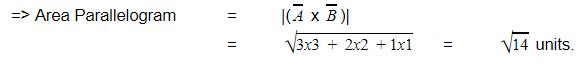QUESTION: 2

### A body is projected horizontally from the top of a cliff with a velocity of 9.8m/s. What time elapses before horizontal and vertical velocities become equal? Take g = 9.8m/s2

Solution:

Horizontal velocity at any instant, vx = u = 9.8m/s
Vertical velocity at any instant, vy = 0 + gt = 9.8t
9.8 = 9.8t
t = 1s

QUESTION: 3

### Two vectors are equal if and only if

Solution:
QUESTION: 4

A cyclist moves along a circular path of radius 70m. If he completes one round in 11s, calculate total length of path.

Solution:

Radius of the circular path, r = 70m
Time takes to complete one round, t = 11s
Total length of the path, s = 2πr = 2*22/7*70 = 440m

QUESTION: 5

Find the odd one out:

Solution:

Force, area and velocity all are a set of different or the same fundamental quantities multiplied or divided together while current itself is a fundamental quantity and can be expressed in terms of any other.

QUESTION: 6

An object thrown from an aeroplane is an example for

Solution:

A projectile is the name given to any body which once thrown into space with some initial velocity, moves thereafter under the influence of gravity alone without being propelled by an engine or fuel. The path followed by a projectile is called its trajectory.

QUESTION: 7

The vector product of parallel vectors is always:

Solution:

The vector product of two vectors depends upon the sine of the angle between the vectors and when the vectors are parallel the sine component would be zero hence the resultant is a null vector.

QUESTION: 8

Given,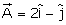and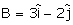. The unit vector of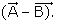is

Solution:
QUESTION: 9

If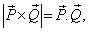what is the angle between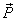and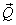Solution:

We know that for two vectors P and Q
P.Q = |P||Q| cos a
And PxQ = |P||Q| sin a
Where a is angle between them
When P.Q = PxQ
We get sin a = cos a
Thus a = 450

QUESTION: 10

A particle of mass 10 g moves along a circle of radius 6.4 cm with a constant tangential acceleration. What is the magnitude of this acceleration, if the kinetic energy of the particle becomes equal to 8 x 10-4 J by the end of the second revolution after the beginning of the motion?

Solution: